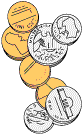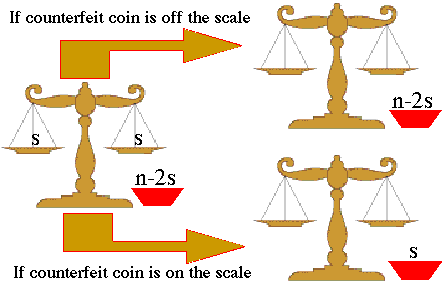The counterfeit Coin Problem

# A DP Perspective on the Counterfeit Coin ProblemPerspective Shmerspective - in this discussion we shall briefly pre-process the problem from a DP point of view before analysing the three versions under consideration.

So we have this collection of N coins and we know that all but one of the coins have the same weight and that the counterfeit coin is say heavier than the other. We have to find the optimal weighing strategy for the identifiction of the counterfeit coin.

The first thing that we do in DP is generalise the problem: how about solving the problems for any number of coins, say n, where n=0,1,2,3,....,N ?

The DP solution will be based on a functional equation relating the optimal solutions of these N+1 problems.

Actually, this is not a bad start because the optimal solutions to four (n=0,1,2,3) of these problems are trivial regardless of the version!

The second thing we do is pretent that we are in the middle of the weighing process and have to decide what to do next. So suppose that we have already conducted a number of weighing and that we contemplate what to do next. The situation is depicted in the figure below:In other words, n coins are yet to be inspected and we are in full control of the situation.

It is clear that we now have to put some coins on the scale. But how many?

This is a good question. The DP answer is something like this: Play the if-then game covering all the feasible (but sensible !) options, and then choose the best option!

So let us apply this recipe:

• Feasibility:
If we have n coins to inspect, then we cannot put on each side of the scale more than Int(n/2) coins, where Int(z) is the integer part of z, eg Int(4.3)=4, Int(5)=5, Int(4.9999)=4. Actually, we can borrow some additional coins from a friend and put more coins on the scale, but this will not be agreeble with the sensibility constraint we discuss below, so let's ignore this option.
• Sensibility:
There is no sense in placing a different number of coins on each side of the scale. It could be a lot of fun, but it will not serve us well in our attempt to speed up the identification of the counterfeit coin.
• If-then game:
Suppose that in the current weighing we decide to place s coins on each side of the scale. How will this influence our next weighing? In particular, how many coins will be left after the current weighing?

The feasibility and sensibility arguments suggest that given that we have n coins to inspect, we should consider placing on each side of the scale s coins, where s=1,2,3,...,Int(n/2). So let

 D(n) := {1,2,3,...,Int(n/2)}

denote the set of feasible decisions we have at this stage of the process. We shall use s to denote these decisions. The situation can be described as follows:In short, if we have n coins to inspect and if we decide to put s coins on each side of the scale, then there will be n-2s coins off the scale, and s must be selected from the set D(n):={1,2,3,...,Int(n/2)}. For example, for n=7 we have D(7)={1,2,3}.

So far so good

The next thing we have to do (a la DP) is examine the impact of the current decision (putting s coins on each side of the scale) on the next stage of the process. This is not difficult because there are only two (relevant) possibilities:

• Either the counterfeit coin will be on the scale (the scale will not be balanced)
• OR it will be off the scale (the scale will be balanced).

In the first case we shall have to inspect s coins and in the second n-2s coins will have to be inspected.

We can describe the process's dynamics by a "if-then" like diagram:As we have already agreed, we shall let Mother Nature, or her loacal agents, decide where the counterfeit coin will end up being. DP will adjust its strategy depending on which agent is handling the case and what objective function is adopted by the agent:

We recommend that you visit these pages in that order. You'll find that the minimax problem is a bit easier than the Expectation problem, and that the Probability problem is much more complicated.